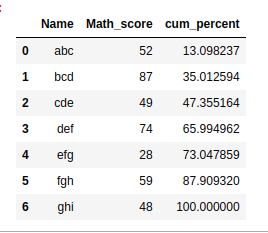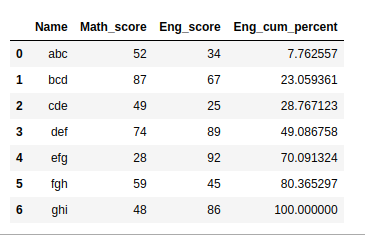Open In App

# Cumulative percentage of a column in Pandas – Python

Cumulative Percentage is calculated by the mathematical formula of dividing the cumulative sum of the column by the mathematical sum of all the values and then multiplying the result by 100. This is also applicable in Pandas Data frames.
Here, the pre-defined cumsum() and sum() functions are used to compute the cumulative sum and sum of all the values of a column.
Syntax:

df[cum_percent] = 100 * (df[‘column_name’].cumsum()/df[‘column_name’].sum())

Example 1:

## Python3

 `import` `pandas as pd``import` `numpy as np`` ` `# Create a DataFrame``df1 ``=` `{``     ``'Name'``:[``'abc'``,``'bcd'``,``'cde'``,``'def'``,``'efg'``,``'fgh'``,``'ghi'``],``   ``'Math_score'``:[``52``,``87``,``49``,``74``,``28``,``59``,``48``]}`` ` `df1 ``=` `pd.DataFrame(df1, columns``=``[``'Name'``,``'Math_score'``])` `# Computing Cumulative Percentage``df1[``'cum_percent'``] ``=` `100``*``(df1.Math_score.cumsum() ``/` `df1.Math_score.``sum``())` `df1`

Output:Example 2:

## Python3

 `import` `pandas as pd``import` `numpy as np`` ` `# Create a DataFrame``df1 ``=` `{``     ``'Name'``:[``'abc'``,``'bcd'``,``'cde'``,``'def'``,``'efg'``,``'fgh'``,``'ghi'``],``   ``'Math_score'``:[``52``,``87``,``49``,``74``,``28``,``59``,``48``],``  ``'Eng_score'``:[``34``,``67``,``25``,``89``,``92``,``45``,``86``]``}`` ` `df1 ``=` `pd.DataFrame(df1,columns``=``[``'Name'``,``'Math_score'``,``'Eng_score'``])` `# Computing cumulative Percentage``df1[``'Eng_cum_percent'``] ``=` `(df1.Eng_score.cumsum() ``/` `df1.Eng_score.``sum``()) ``*` `100` `df1`

Output: